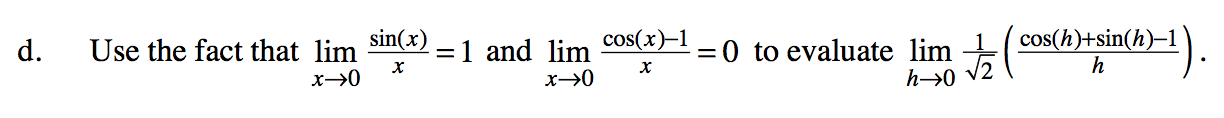### Home > PC3 > Chapter 8 > Lesson 8.3.4 > Problem8-144

8-144.
1. Let f(x) = sin(x). Homework Help ✎

1. Calculate the average rate of change from x =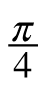to x =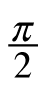.

2. Write an expression for the average rate of change from x =to x =+ h.

3. Use the fact that sin(A + B) = sin(A)cos(B) + cos(A)sin(B) to show that the expression you wrote in part (b) can be rewritten as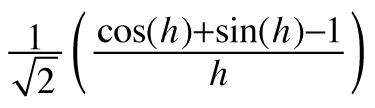.

4. Use the fact that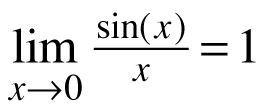and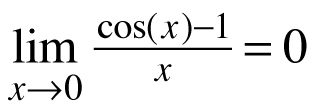to evaluate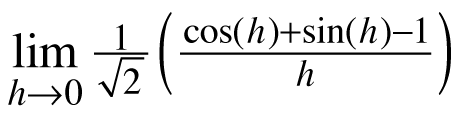.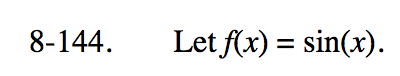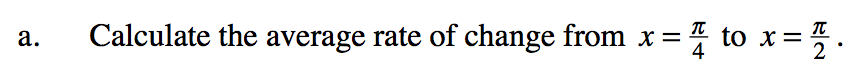$\frac{s\left(\frac{\pi}{2}\right)-s\left(\frac{\pi}{4}\right)}{\left(\frac{\pi}{2}\right)-\left(\frac{\pi}{4}\right)}$$\frac{s\left(\frac{\pi}{4}+h\right)-s\left(\frac{\pi}{4}\right)}{\left(\frac{\pi}{4}+h\right)-\left(\frac{\pi}{4}\right)}$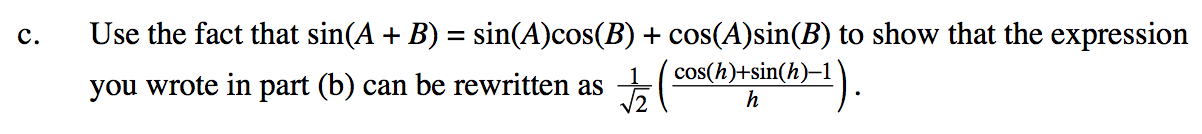$m=\frac{\text{sin}\left ( \frac{\pi }{4}+h \right )-\text{sin}\left ( \frac{\pi }{4} \right )}{h}=\frac{\text{sin}\left ( \frac{\pi }{4}\right )\text{cos}(h)+\cos\left ( \frac{\pi }{4} \right )\text{sin}(h)-\text{sin}\left ( \frac{\pi }{4} \right )}{h}$

Substitute values for the trigonometric expressions and simplify.